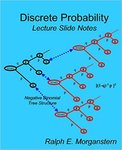## Faculty Book Gallery#### Title

Discrete Probability:

#### Description

These Lecture Slide Notes have been used for a two-quarter graduate level sequence in probability covering discrete and continuous probability in two separate volumes. Although reasonably self-contained, they do not constitute a formal exposition on the subject; rather the intent is to provide a concise and accessible format for reference and self-study. In this regard, each slide stands alone to encapsulate a complete concept, algorithm, or theorem, using a combination of equations, graphs, diagrams, and comparison tables. The explanatory notes are placed directly below each slide in order to reinforce key concepts and give additional insights. A Table of Contents serves to organize the slides by topic and gives a complete list of slide titles and their page numbers. An index is also provided in order to link related aspects of topics and also to cross-reference key concepts, specific applications, and the abundant visual aids.

This book constitutes the first volume on discrete probability; a second volume will cover continuous probability. Part 1 covers counting with and without replacement, axiomatic probability models, computation techniques, conditional, joint, marginal, and Bayesian update probabilities. The concept of a random variable (RV) is fully characterized by a discrete probability mass function (PMF) and a quasi-continuous cumulative distribution function (CDF). A numerical characterization of a RV is given by the mean, variance, and expectation value. Pairs of RVs give way to new concepts such as independence, covariance, and the effects of linear and bi-linear transformations. Common discrete probability mass functions (PMFs) are discussed in terms of related pairs, tree diagrams, and algebraic representations.

2013

#### Publisher

CreateSpace Independent Publishing Platform

#### Disciplines

Probability | Statistics and ProbabilityCOinS